# PLC Logic – The Logic Behind The PLC Program

This is an introduction to PLC logic. You can use PLC logic to build PLC programs with simple electrical circuit diagrams. In this article, I will use combinatorial logic to build the functionality of a PLC program.

And to get as close as possible to real a PLC programming language like ladder logic, the PLC logic in this article will be contacts and relays in an electrical circuit.

The first PLC program you will be building will be a very simple one. You will be using combinatorial logic to build your PLC program.

The reason for this is that a PLC program is really just logic. Everything in a PLC has two states, just like in digital logic. In logic, the two states are called FALSE and TRUE. Sometimes these two states are referred to as “0” and “1”.

Those two states are referred to as the numbers 0 and 1, so that we can use them in binary numbers.

Since the PLC works with two states only, it makes sense to use the base-2 numeral system or binary numbers to represent the two states. Binary numbers, just like the PLC, has two numbers or states. 0 and 1. We can now represent the FALSE with 0 and TRUE with 1. This will be very helpful when working with analog inputs, outputs and calculations.

The PLC uses logic to decide how the outputs will be set. The inputs of all this logic will represent the PLC inputs. All the logic will be our PLC program. At last, the outputs of the logic will be the outputs of our PLC program.

## Your First PLC Logic Program

Now, let us get started by making a very simple imaginary PLC program with PLC logic. The functionality of this program is simply to take the status of one input and invert it to one output.

So that your first logic program will function like this:

• If the input is 0 or FALSE; then the output will be 1 or TRUE.
• If the input is 1 or TRUE; then the output will be 0 or FALSE.

Let us put the functionality into the scan cycle of the PLC:

1. Scan the inputs.
2. Use the input I0.0 and set output Q0.0 as the inverted.
3. Set the outputs

The first step in the scan cycle is the PLC scanning the states of all its inputs. The second step will be our logic PLC program, represented by logic gates. This is where the logic happens and where the state of the output will be decided by the state of the input and out logic.

For now, do not worry about the specific names of the input and output used in this example. I chose to call the input I0.0 because in a Siemens PLC like the Siemens S7-1200, this is the naming convention for inputs. The same applies to the name of the output Q0.0.

Each PLC manufacturer has its own naming convention for the inputs and outputs, and this depends on how many inputs and outputs the PLC has. If you want to know more about I/O addressing the Siemens PLC check out the S7-300 instruction list page 7-10. For Siemens, the inputs have the letter I, and the address, and the letter Q for outputs.

## Making Use of PLC Logic

Now that you know about the inputs, outputs, and functionality, you can begin to build the PLC logic:

You have to use input I0.0 to set output Q0.0, so a natural start would be to connect the input and the output directly: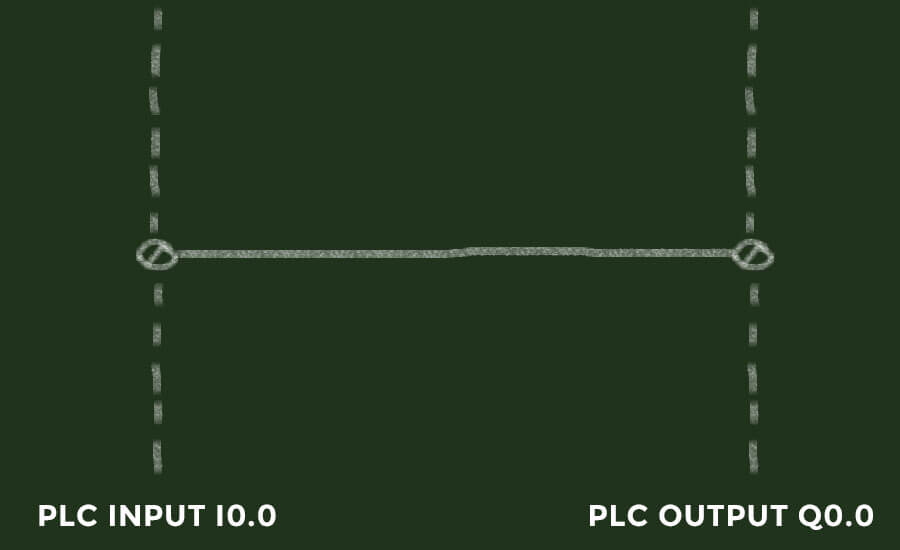Direct connection between input and output in PLC logic

But this would only mean that your output will have the same state as your input. There is no need to look at the truth table for this. When the input is 0 then output is 0, and when the input is 1, the output is 1.

In some way, the state of the output has to be the inverted input. You have to use the NOT-gate or the inverter to invert the output. The function of the NOT-gate is to invert the input signal to its output.

Here is how that will look:NOT-gate to illustrate the inverter function in PLC logic.

This would invert the input, and then send it to the output. Just like the program should work. You can even look at the truth table for this simple program, and see that the output is the inverted input:

 I0.0 Q0.0 0 1 1 0

The example here is very simple and just to illustrate how a PLC program really works. A PLC program is the logic that decides the states of the outputs. You can then choose to use the inputs in your logic so they will, with your logic, decide the states of the outputs.

Even though many PLC’s have communications and other advanced functions, the main functionality of the PLC is always to decide the states of the outputs with logic.

## Representing PLC Logic with Electrical Circuits

This PLC logic program you just saw with the NOT-gate is still far away from how PLC programming looks. So in order to get a little closer to real PLC programming, in this case, ladder logic, we have to draw this PLC logic program in another way.

The PLC programming language ladder logic is very visually similar to electrical circuit diagrams. You can take advantage of this similarity by thinking about the PLC logic with contacts and relays. Because, as I explained in my article about combinatorial logic, contacts and relays both have two states. Just like the PLC logic. A contact can have the two states ON or OFF. The same can a relay.

By using normally open and normally closed contacts in a combination with relays, you can create a piece of logic very similar to real PLC programming. Let me explain that further by using the above example with the NOT-gate.

### Contacts in PLC Logic for Inputs

In the above example, I used a NOT-gate to illustrate the inverted logic of the PLC logic program. The NOT-gate is also called the inverter function can be illustrated with a normally closed contact. The normally closed (NC) contact is in PLC programming referred to as an examination if open instruction (XIO). Examine if closed is an instruction that examines a bit for the off condition.

To illustrate that with the normally open contact, you can think of the two sides of the contact as an input and an output. When the input of the contact is off (open), then the output of the contact will be on. Or said in another way; the output will examine a bit. And thereby the function name examines if open.

Here is how the illustration with the normally open contact looks like: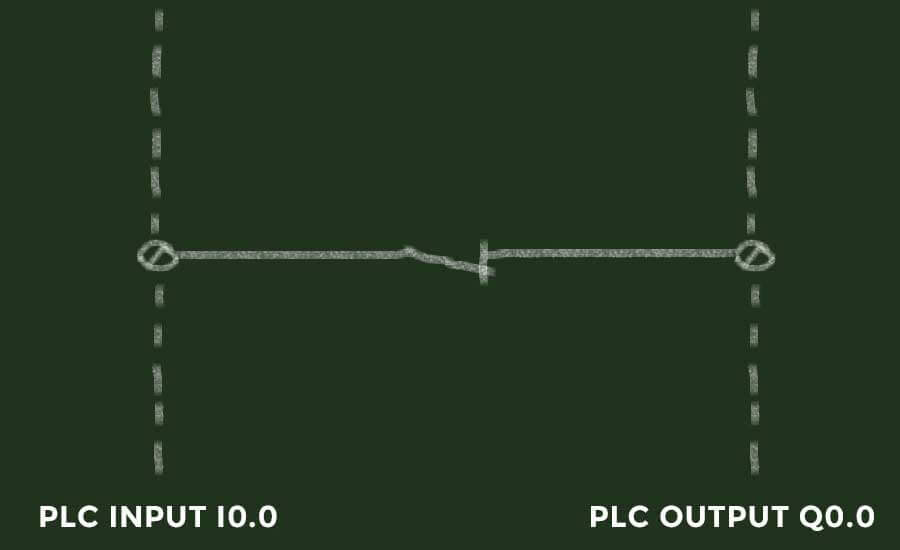Normally open contact to illustrate examine if open PLC logic.

The normally closed contact will now represent the PLC logic. And still, the functionality will be the same as before. As long as the input I0.0 is off, the output Q0.0 will be on. The exact same function as the NOT-gate.

What we are really doing here, is that we are not only representing PLC logic with contacts. We are representing the inputs with the contacts. So that in our PLC logic, the contacts will represent the logic and the state of the contacts will represent the state of the inputs.

Be aware that the contacts used to represent the inputs are not the same as the actual physical contacts, connected to the PLC inputs. The contacts used to represent inputs are really just boolean instructions. You can read about the difference between the boolean instructions and physical contacts in my article about the normally closed stop button.

#### Negative Logic

In this example, the input I0.0 is represented by the normally closed contact. By doing so, we are making what is also called negative logic. Negative logic is the case when the on and off states are switched.

Said in another way: when the state of the input is off, the logic state is on. And when the state of the input is on, the logic state is off.

Here is a video that explains the negative logic, which by the way is the same as the examine if open instruction I mentioned before:

### Representing PLC Outputs with Relays

In PLC programming and therefore in PLC logic we do not only represent the inputs. The outputs have to be represented too. They have to be represented in a way so that we can illustrate two states. Because as with the inputs, the digital outputs of a PLC can only have two states. On or off.

Since we are already using electrical circuits to illustrate the inputs, why not keep on that track. To represent the outputs we will be using relays. There is two reasons to use relays to represent PLC outputs:

1. Relays have two states like the PLC outputs
2. Relays have functional contacts to use for latching and much more

So, keeping in mind that each PLC output is now a relay, we could continue with the PLC logic example.

This is how the illustration of the PLC logic program now looks like: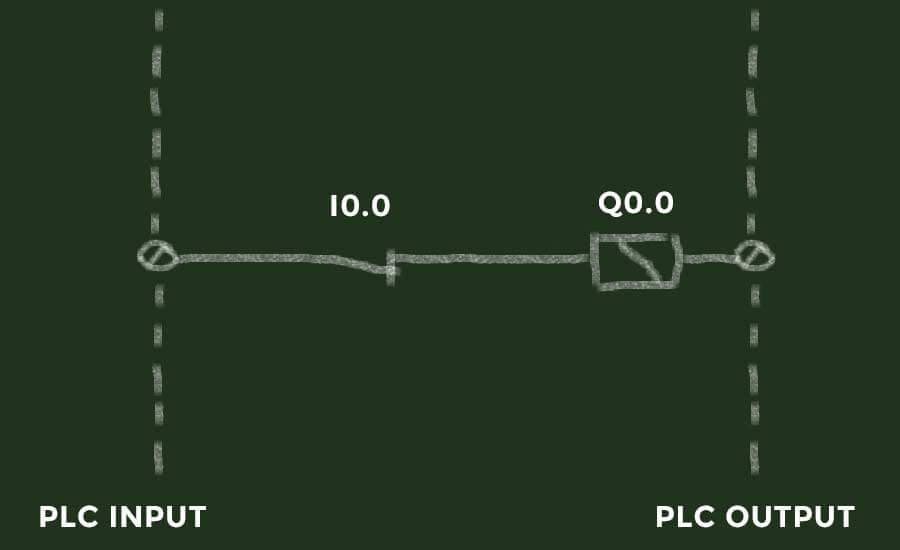Representing the PLC output with a relay.

Now the output Q0.0 is represented by a relay. Or to be more precise the state of the output is now represented with a relay. When the relay in our PLC logic is on the state of the output will also be on.

Another great feature of relays is functional contacts. For each relay, you can have several normally open or normally closed contacts representing the state of the relay. The functional contacts will change state with the relay. One of the most common uses of functional contacts on relays is latching.

Latching is the act of keeping the relay energized or on by using a functional normally open contact on the same relay. This can be done in PLC logic as well to keep a PLC output on.

Take a look at this illustration. This time the PLC input I0.0 is represented with one normally open contact but the PLC output is now represented by both a relay and a contact.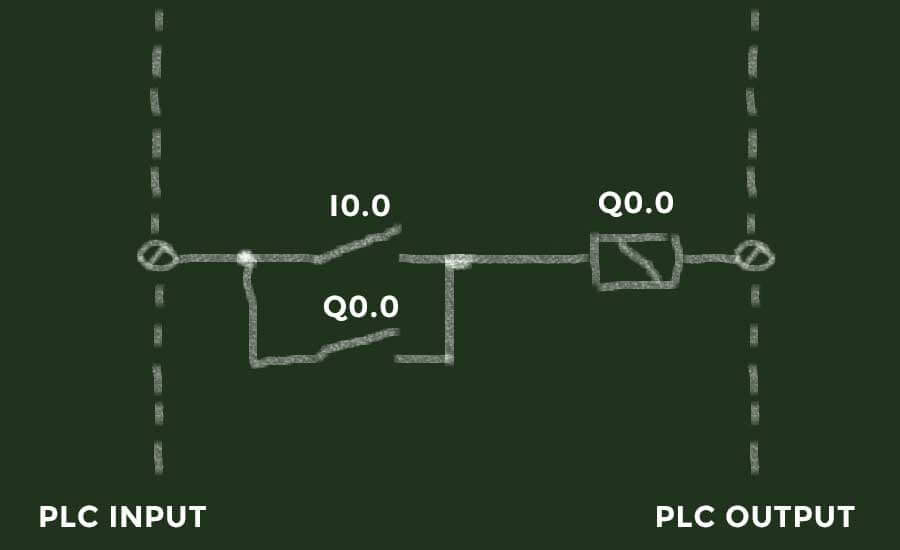Latching a relay or a PLC output with a normally open contact.

When the state of the input I0.0 is 1, the state of the normally open contact I0.0 will be on. The relay will be energized and the PLC output Q0.0 will be on. What happens next is latching.

Now that the state of the output has changed to 1, the state of the normally open contact representing the output will change to on. This means that even though the state of the PLC input should change to 0, the relay will still be energized. The relay or the output Q0.0 will be energized by itself.

Be aware that this is just an example. In this example, it would be impossible to de-energize the relay and turn the output off. To do so, you would have to insert another contact between the output contact and the output relay to break the connection. For example, you could use another input represented by a normally closed contact: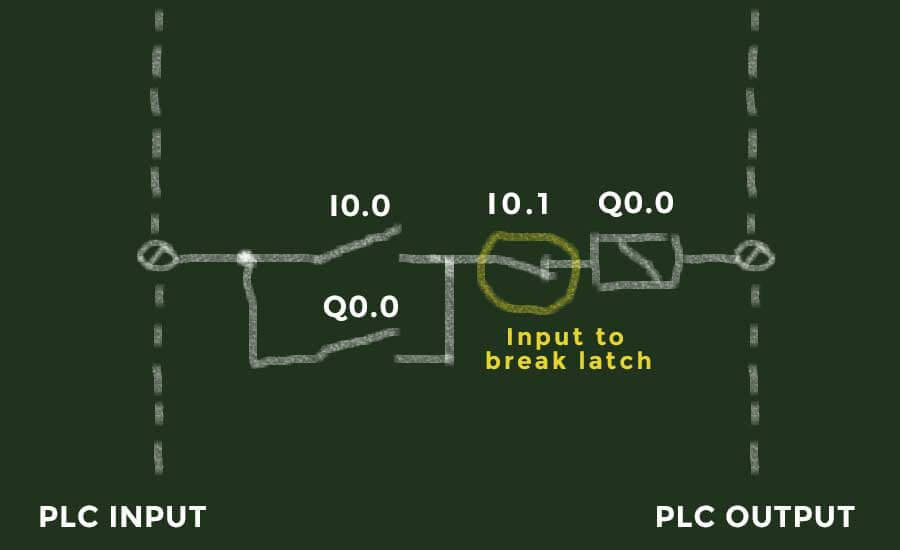Latching a PLC output and breaking the latch with a normally closed contact.

We can sum all the PLC logic up to this:

• The state of the input is set outside the PLC logic and represented by contacts
• The state of the outputs is set inside the PLC logic and represented by relays and contacts

I would recommend reading a good PLC programming book to explore this in further detail.

### Electrical Circuit Diagrams and PLC Programming

It looks pretty much like an electrical circuit diagram, and it certainly almost is. You only have to think about the input side as the positive side of a power source and the output side as the negative or ground side.

There is a special reason for doing this. Actually, there are two reasons, but let me start with the obvious reason:

The PLC programming language called ladder logic looks almost like electrical circuits.

Take a peek at this small piece of ladder logic: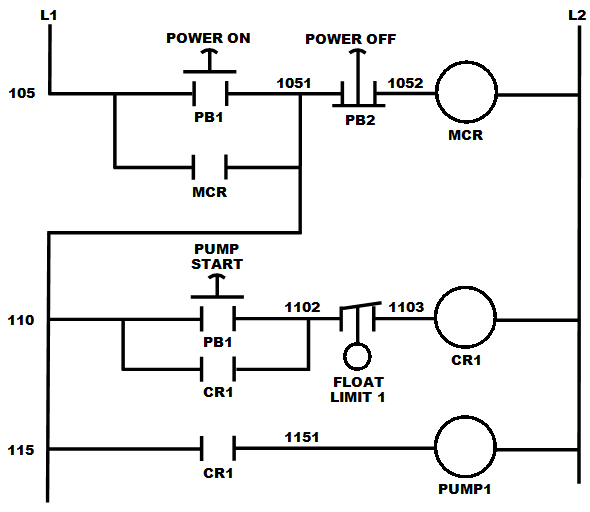The ladder logic diagram looks just like an electrical circuit diagram. Source: Wikipedia

It looks a lot like an electrical circuit, and the functionality of ladder logic is just as described in this article. Ladder logic is the most visual representation of PLC logic. For most beginners ladder logic is the way to do PLC programming.

Other PLC programming languages are available, but I think ladder logic and ladder diagrams are the most well known. The ladder is also the easiest way to get into PLC programming.

You should now have a better understanding of how PLC logic works. And if you haven’t started PLC programming yet, you should be ready to start learning ladder logic programming.

•